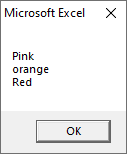# VBA For Each Loop

For Each loop is used to execute a statement or group of statements for each element in an array.

For Each” loop is similar to the “For” loop. This loop is executed for each element in an array. Therefore, the step counter will not exist in this loop. It is commonly used with arrays or in the context of the File system objects to operate recursively.

### Syntax

You can build a cycle through a set of collections using the “For Each” loop in VBA.

Here are some examples of a set of collections using the For Each loop in the excel VBA, such as:

• A group of all the open workbooks.
• A group of all the worksheets in the workbook.
• A group of all the cells in the range of selected cells.
• A group of all the charts or shapes in the workbook.

Using the “For Each” loop, you can go through each of the objects in the collections, and also perform some operations on it.

For example, You can go through all worksheets in the workbook and protract these worksheets. Or you can go through all the cells in the worksheet and change the formatting also.

With the For Each loop, you don’t need to know how many objects are there in the collection.

For Each loop automatically go through each object and perform the specified operations.

For example, If you protect all the worksheets in a workbook, the code will be the same whether you have a workbook with 1 worksheets or 20 worksheets.

### Example

Let’s suppose you have a workbook where you want to protect all the worksheets. Such as:

In the above example, we used the ‘ws‘ variable as a Worksheet object. Which tells to VBA that ‘ws‘ should be used to interpret as a worksheet object in that code.

Now we use the “For Each” statement to go through each “ws” in the collection of the worksheets in the active workbook.

Note that unlike other loops where you tried to protect all the worksheets in a workbook, here you don’t need to worry about how many worksheets are there in the workbook.

You don’t need to count these to run the loop. For Each loop ensures that all the objects are analyzed one by one.

Now, to go through all the open workbooks and save all:

If you are working with multiple workbooks at the same time, it can be able to save all these workbooks at once. Such as,

Note that in the above example, to save the workbook in a specific location, you don’t get a prompt that asks you in this case. It saves it in the default folder.

### ExampleExecutes the above code, you will get the following output, such as:Next TopicVBA Do While Loop